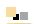# void star games

* play * code * learn *

##Flash Memory Game Tutorial 3 BackStart OverNext

A New Class. Create a new Actionscript file and call it Table.as. Inside declare a package and then a class called Table. Copy the import files from the class Tile into this new one.

First, let us declare a variable in this class to store the table of tiles. This will have to be an array and we can make it one right away:
var table:Array;
Declaring the type of a variable of parameter in Flash doesn't affect the program but Flash can help you finding appropriate functions for it if you do.

Now we can define a function that initializes the tiles with random values such that there are two and only two tiles for each option. The easiest way is to fill the table in order and then shuffle it. Let's start with the function shuffle:
public function shuffle(rows:int, cols:int) {
var i, r, temp;
for (i=0; i<rows*cols-1; i++) {
r = i+Math.floor(Math.random()*(rows*cols-i));
if (i != r) {
temp=table[int(i/cols)][i%cols];
table[int(i/cols)][i%cols] = table[int(r/cols)][r%cols];
table[int(r/cols)][r%cols] = temp;
}
}
}

Basically this function chooses for each cell in the table a random position further down in the table and swaps it with it. I find it easier to linearize the table by having i going from 0 to rows*cols-1 and then compute the row and column by dividing by the number of columns and then applying the modulo (%) operation.

Now that we have a shuffle function, we can write the function that fills up the table. Here it is:
public function fill_table(rows:int, cols:int, options:int) {
var k = rows*cols/2;
var n = options;
var i=0, opt = 0;
while (k > 0) {
var r = Math.floor(Math.random() * (1+n));
if (r <= k) {
table[int(i/cols)][i%cols] = opt;
table[int((i+1)/cols)][(i+1)%cols] = opt;
i+=2;
k--;
}
opt++;
n--;
}
shuffle(rows, cols);
}

What happens here is that we go through all the possible options and we select rows*cols/2 of them to be used in the table with a known sample selection algorithm. For each selected option we need to place two tiles with that option in the table. Again, I found it easier for this algorithm to work with a linearized table and compute the row and column once I know what value needs to be stored in the cell.

Finally, if you want to test out this part (and you should), here's a function that can be useful:
public function trace_table(rows, cols) {
for (var i=0; i<rows; i++) {
for (var j=0; j<cols; j++) {
trace(i, j, table[i][j]);
}
}
}

The "trace" is printing out the parameters it receives to the Output tab in flash. It does not affect the execution of the swf, but it's useful for debugging.

With all of this, it's time to write a constructor for the class. It seems necessary to pass the number of rows and columns to the constructor, but also to store these in some class variables for later use. Let's add two variables in the class called sizeR and sizeC (or use more creative names if you want). This constructor must initialize the array of arrays that will store the table, then call the function fill_table. We can assume right now that the constructor will need to initialize the tile objects and keep links to them, in which case it will need a reference to the stage that it can pass to the tiles.
public function Table(rows:int, cols:int, the_stage) {
table = new Array(rows);
for (var i=0; i<rows; i++) {
table[i] = new Array(cols);
}
fill_table(rows, cols, 15);
sizeR=rows;
sizeC=cols;
parent = the_stage;
}

Before going to the next step, it might be a good idea to test this all out in the .fla file.

Let's proceed to creating the tiles and displaying them. We'll need another array created the exact same way as the table. Let's call it "tiles" and add it to the class. First, let's assume that we'll leave some space in the stage above the rows of tiles for buttons and titles. It would be useful to store it in a class variable. Declare it as "headspace" and initialize it in the constructor to whatever might be appropriate for you. You can make a guess now and adjust this value later by testing it.

Then start a new function called create_tiles with no parameters and allocate the arrays for the tiles with a similar method to the one used for the table. Instead of the parameter "rows" you'll have to use sizeR this time, and instead of "cols", sizeC. Then inside the for loop, after initializing the array for the row i, let's add a loop that creates all the tiles for that row and places them in the appropriate position ("this" identifies the table object itself):
for (var j=0; j<sizeC; j++) {
tiles[i][j] = new Tile(parent, table[i][j]);
tiles[i][j].set_position(i, j, this);